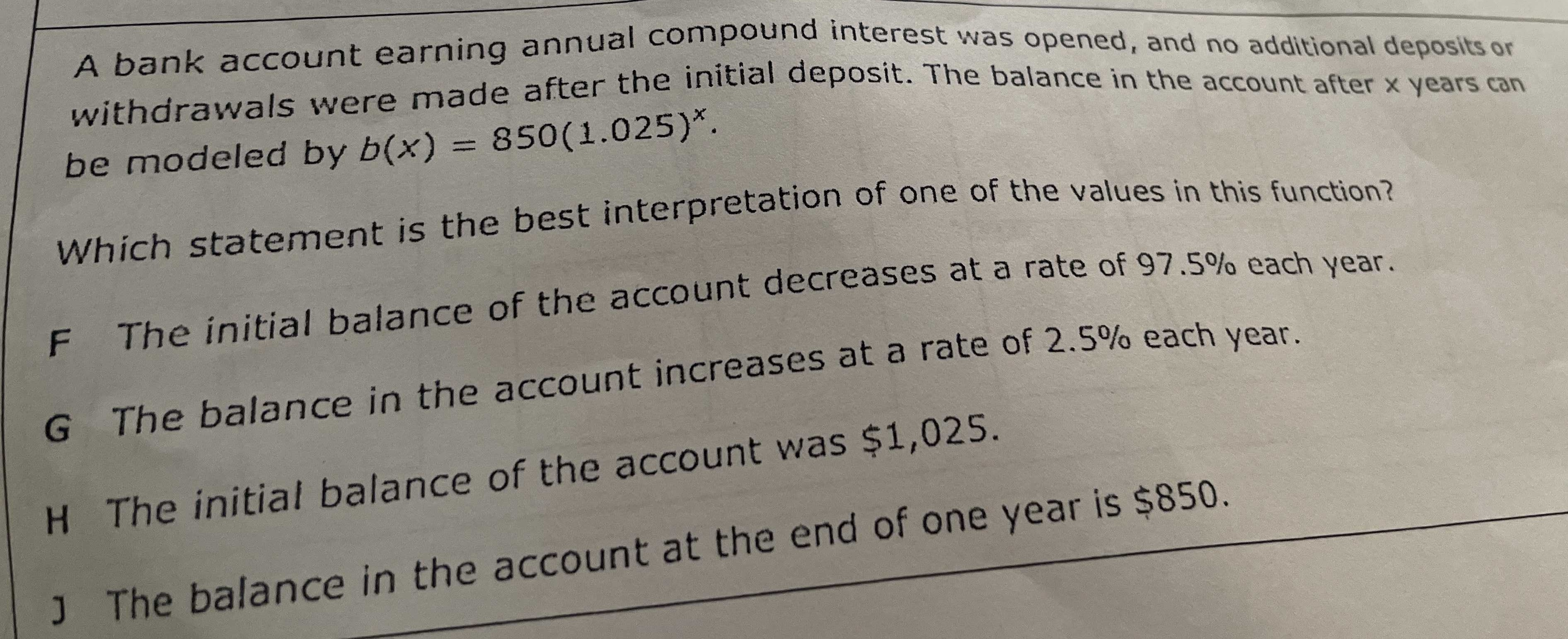### ¿Todavía tienes preguntas de matemáticas?

Pregunte a nuestros tutores expertos
Algebra
Pregunta

A bank account earning annual compound interest was opened, and no additional deposits or withdrawals were made after the initial deposit. The balance in the account after $$x$$ years can be modeled by $$b ( x ) = 850 ( 1.025 ) ^ { x }$$ .

Which statement is the best interpretation of one of the values in this function? F The initial balance of the account decreases at a rate of $$97.5 \%$$ each year. G The balance in the account increases at a rate of $$2.5 \%$$ each year. H The initial balance of the account was $$\ 1,025$$ . J The balance in the account at the end of one year is $$\ 850$$ .